# Intermediate Algebra Tutorial 34

Intermediate Algebra
Tutorial 34: Complex Fractions

WTAMU > Virtual Math Lab > Intermediate AlgebraLearning Objectives

After completing this tutorial, you should be able to:
1. Simplify complex fractions.Introduction

Are fractions getting you down?  If so, you are not alone.  Well, you are in luck. We have one more tutorial devoted to rational expressions (fractions).  In this tutorial we will be looking at simplifying complex fractions.  If you need a review on simplifying and dividing rational expressions, feel free to go back to Tutorial 32: Multiplying and Dividing Rational Expressions. If you need a review on finding the LCD of fractions feel free to go back to Tutorial 33: Adding and Subtracting Rational Expressions. It is time to get started with this tutorial.Tutorial

Complex Fraction

A complex fraction is a rational expression that has a fraction in its numerator, denominator or both.

In other words, there is at least one small fraction within the overall fraction.

Some examples of complex fractions are: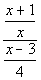and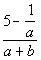There are two ways that you can simplify complex fractions.  We will call them method I and method II.

Method I
Simplifying a Complex Fraction

In other words, you will be combining all the parts of the numerator to form one fraction and all of the parts of the denominator to form another fraction.  If you need a review on adding and subtracting rational expressions, go to Tutorial 33: Adding and Subtracting Rational Expressions.

Step 2:  Divide the numerator by the denominator by multiplying the numerator by the reciprocal of the denominator.

Step 3: If needed, simplify the rational expression.

If you need a review on simplifying rational expressions, go to Tutorial 32: Multiplying and Dividing Rational Expressions.Example 1: Simplify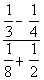.

Step 1:   If needed, rewrite the numerator and denominator so that they are each a single fraction.

Combining only the numerator we get: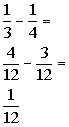*Rewrite fractions with LCD of 12

Combining only the denominator we get: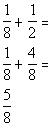*Rewrite fractions with LCD of 8

Putting these back into the complex fraction we get: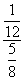*Write numerator over denominator

Step 2:  Divide the numerator by the denominator by multiplying the numerator by the reciprocal of the denominator

AND

Step 3: If needed, simplify the rational expression.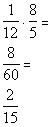*Rewrite div. as mult. of reciprocal

*Divide out a common factor of 4Example 2: Simplify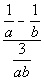.

Step 1:   If needed, rewrite the numerator and denominator so that they are each a single fraction.

Combining only the numerator we get: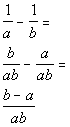*Rewrite fractions with LCD of ab

The denominator is already written as one fraction: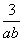Putting these back into the complex fraction we get: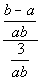*Write numerator over denominator

Step 2:  Divide the numerator by the denominator by multiplying the numerator by the reciprocal of the denominator

AND

Step 3: If needed, simplify the rational expression.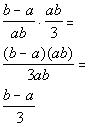*Rewrite div. as mult. of reciprocal

*Divide out common factors of a and b

Method II
Simplifying a Complex Fraction

Step 1: Multiply the numerator and denominator of the overall complex fractions by the LCD of the smaller fractions.

Recall that when you multiply the exact same thing to the numerator and the denominator, the result is an equivalent fraction.  If you need a review on finding the LCD, go back to Tutorial 33: Adding and Subtracting Rational Expressions.

Step 2: If needed, simplify the rational expression.Example 3: Simplify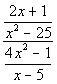.

Step 1: Multiply the numerator and denominator of the overall complex fractions by the LCD of the smaller fractions.

The denominator of the numerator’s fraction has the following two factors: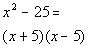The denominator of the denominator’s fraction  has the following factor: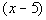Putting all the different factors together and using the highest exponent, we get the following LCD for all the small fractions: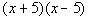Multiplying numerator and denominator by the LCD we get: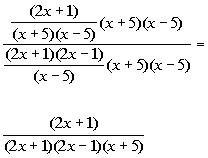*Mult. num. and den. by (x + 5)(x - 5)

Step 2: If needed, simplify the rational expression.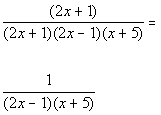*Divide out the common factor of (2x + 1)Example 4: Simplify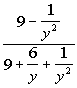.

Step 1: Multiply the numerator and denominator of the overall complex fractions by the LCD of the smaller fractions.

The denominator of the numerator’s fraction has the following factor: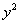The denominator of the denominator’s fraction  has the following factors:

y andPutting all the different factors together and using the highest exponent, we get the following LCD for all the small fractions:Multiplying numerator and denominator by the LCD we get: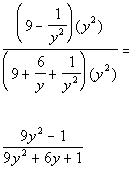*Mult. num. and den. by y squared

Step 2: If needed, simplify the rational expression.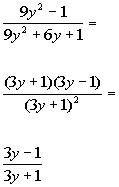*Num. factors as a difference of two squares
*Den. factors as a perfect square trinomial.

*Divide out the common factor of (3y + 1)Example 5: Simplify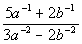.

At first glance, this does not look like a complex fraction. However, once you rewrite it with positive exponents you will see that we really do have a complex fraction.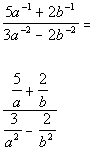*Rewrite with positive exponents

Step 1: Multiply the numerator and denominator of the overall complex fractions by the LCD of the smaller fractions.

The two denominators of the numerator’s fractions have the following factors:

a and b

The two denominators of the denominator’s fractions  have the following factors: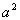and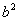Putting all the different factors together and using the highest exponent, we get the following LCD for all the small fractions: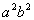Multiplying numerator and denominator by the LCD we get: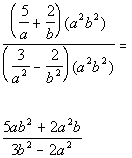*Mult. num. and den. by a squared b squared

Step 2: If needed, simplify the rational expression.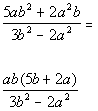*Factor out the GCF of ab in the num.Practice Problems

These are practice problems to help bring you to the next level.  It will allow you to check and see if you have an understanding of these types of problems. Math works just like anything else, if you want to get good at it, then you need to practice it.  Even the best athletes and musicians had help along the way and lots of practice, practice, practice, to get good at their sport or instrument.  In fact there is no such thing as too much practice.

To get the most out of these, you should work the problem out on your own and then check your answer by clicking on the link for the answer/discussion for that  problem.  At the link you will find the answer as well as any steps that went into finding that answer.Practice Problems 1a - 1b: Simplify.

1a.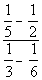1b.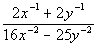Need Extra Help on these Topics?

Go to Get Help Outside the Classroom found in Tutorial 1: How to Succeed in a Math Class for some more suggestions.Last revised on July 17, 2011 by Kim Seward.